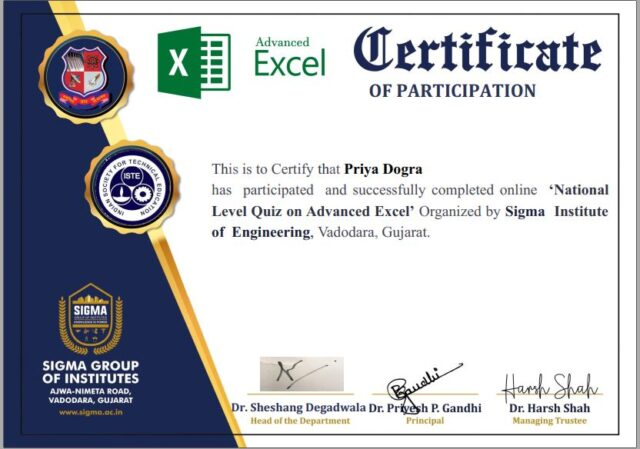Home Certification Microsoft Excel Free Certification | Advance Excel Free Certificate

# Microsoft Excel Free Certification | Advance Excel Free Certificate

0Q1) Functions in MS Excel must begin with _ *

• A Plus Sign
• A > Sign
• An () sign
• An Equal Sign

Q2) Which one is not a Function in MS Excel ? *

• MIN
• MAX
• AVG
• SUB

Q3) Which function in Excel tells how many numeric entries are there ? *

• COUNT
• SUM
• NUM
• CHKNUM

Q4) Which function in Excel checks whether a condition is true or not ? *

• AVERAGE
• IF
• SUM
• COUNT

Q5) How many sheets are there, by default, when we create a new Excel file ? *

• 3
• 10
• 5
• 1

Q6) MS-Excel uses the . . . . Function when creating a data table *

• Table
• Count
• Both (a) & (b)
• Average

Q7) In MS-Excel which of the following is an absolute cell reference? *

• None of these
• !A!1
• \$A\$1
• #a#1

Q8) Data Validation allows you to stipulate what type of data is allowed within a cell. Which of the following is NOT a valid Validation Criteria type? *

• Images or Shapes
• Whole Numbers
• Date and Time
• Months of the Year

Q9) What MS-Excel feature can you use if you want to work with one record at a time? *

• Auto Complete
• Auto Filter
• Data Form
• All of the above

Q10) In MS-Excel what does SUMIF function do? *

• Adds all the numbers in a range of cells
• Returns a subtotal in a list or database
• Adds up cell values based on a condition